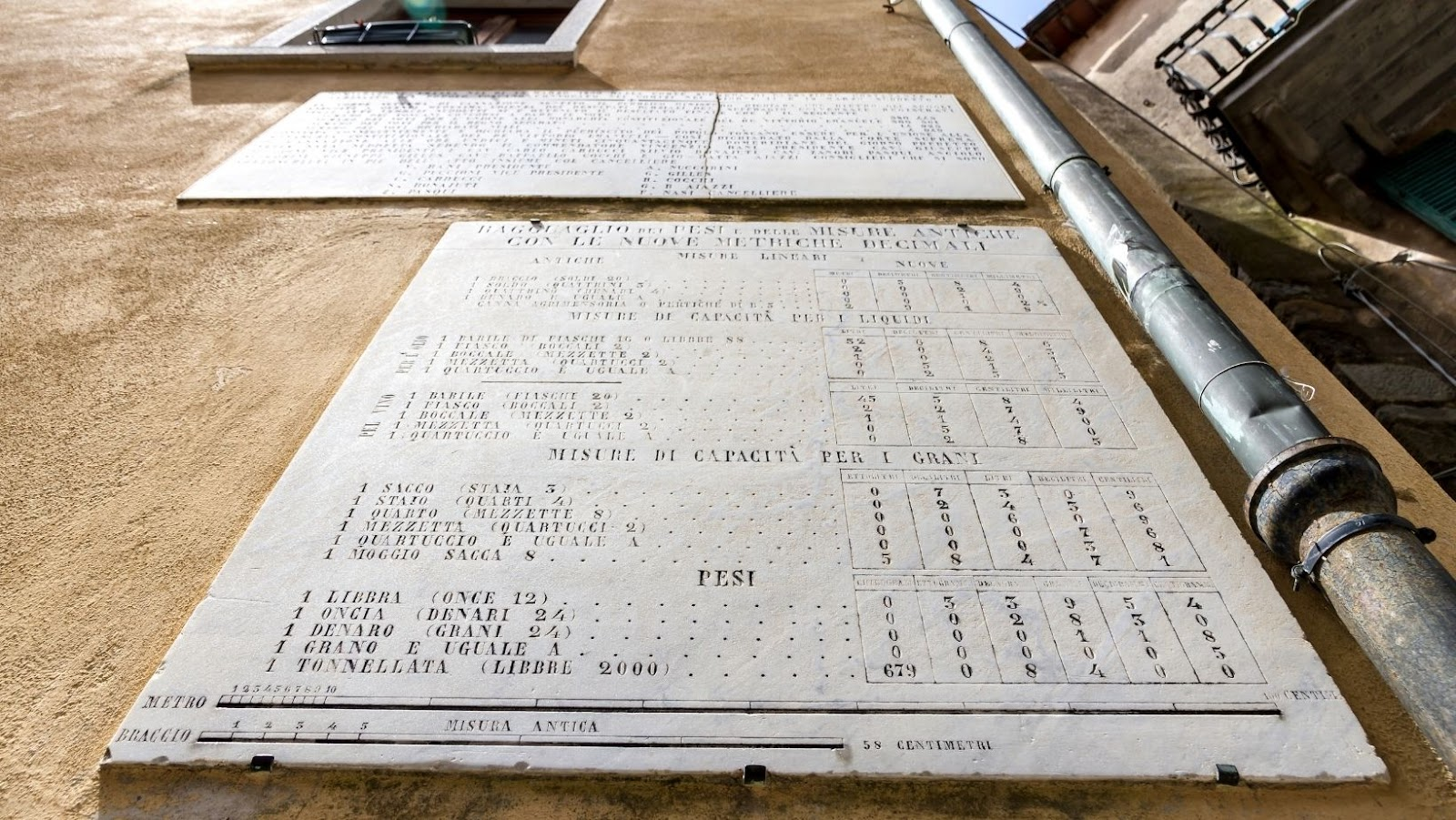## What is the Metric system and how did it come to be used around the world

The metric system is a decimalized system of measurement that was first developed in France. It is now used around the world. The metric system is based on base units and prefixes. The first practical realisation of the metric system came in 1799, during the French Revolution, after the existing system of measures had become impractical for trade, and was replaced by a decimal system based on the kilogram and the metre. The basic units were taken from the natural world.

## How do you measure things in the Metric system

The base unit for length is the meter, for mass is the kilogram, and for time is the second.

## Advantages of using the Metric system over other measurement systems

The advantages of using the metric system over other measurement systems are as follows:

• The metric system is more accurate than other measurement systems.
• The metric system is more efficient than other measurement systems.
• The metric system is more logical than other measurement systems.

## Disadvantages of using the metric system

While the metric system does have many advantages over other measurement systems, it does have a few disadvantages as well.

Some people argue that the metric system is less intuitive than other measurement systems. It can be confusing to learn all of the base units and prefixes.

Others argue that the metric system is more accurate and efficient than other measurement systems. And, once you learn the basics of the metric system, it is just as easy (if not easier) to use than any other measurement system.

## Imperial system

The Imperial System is also called The British Imperial because it came from the British Empire that ruled many parts of the world from the 16th to the 19th century. After the U.S. gained independence from Britain, the new American government decided to keep this type of measurement, even though the metric system was gaining in popularity at the time. We are one of the few countries in the world that still use this system, and first-time visitors can find it confusing.

## How do you measure things in The Imperial system

The Imperial system of measurement uses units such as feet, inches, pounds, and gallons.

## Comparison in measurements between The Metric system and The Imperial system

• 1 mile equals 1.6 Kilometers
• 1 foot (12 inches) is equal to 30 centimeters
• 1 inch is about 25 millimeters or 2.54 centimeters
• A 3-foot measurement is almost exactly 1 meter
• 1 Kilogram is just over 2 pounds
• 1 pound is about 454 grams
• For U.K. visitors, 14 pounds = 1 stone

## How many inches is 44mm

44mm is equal to 1.73 inches.

There is no one definitive conversion formula for converting mm to inches, as there are different ways to do the conversion. One possible formula is:

(mm/100) x (inches/2.54).

How many kilometars equals 3 miles?

There are 1.6 kilometers in 3 miles.

To convert kilometers to miles, divide the kilometers by 1.6.

To convert miles to kilometers, multiply the miles by 1.6.

How many pounds are in 13kg?

There are 13 kg in 28.35 pounds.

To convert kg to pounds, divide the kg by 2.2046.

To convert pounds to kg, multiply the pounds by 0.45359.

## Celsius vs Fahrenheit

The Celsius scale is a measurement system used to measure temperature. It is named after Anders Celsius, a Swedish scientist who created the scale in 1742. The Fahrenheit scale is another measurement system used to measure temperature. It is named after Daniel Gabriel Fahrenheit, a German scientist who created the scale in 1724.

## How do you convert temperatures from one scale to the other

To convert temperatures from one scale to the other, you use the following formula:

Celsius = (Fahrenheit – 32) / 1.8

Fahrenheit = (Celsius * 1.8) + 32

• 0 degrees Celsius is equal to 32 degrees Fahrenheit. (The freezing point)
• 24 degrees Celsius is equal to 75 degrees Fahrenheit. (A very pleasant day)